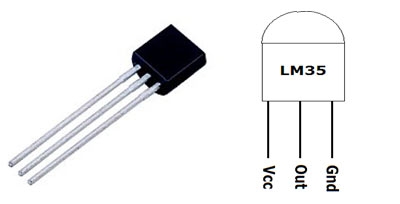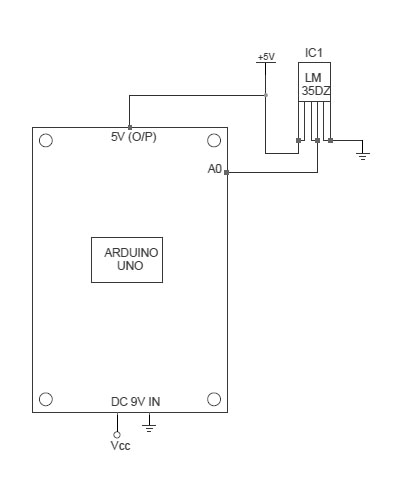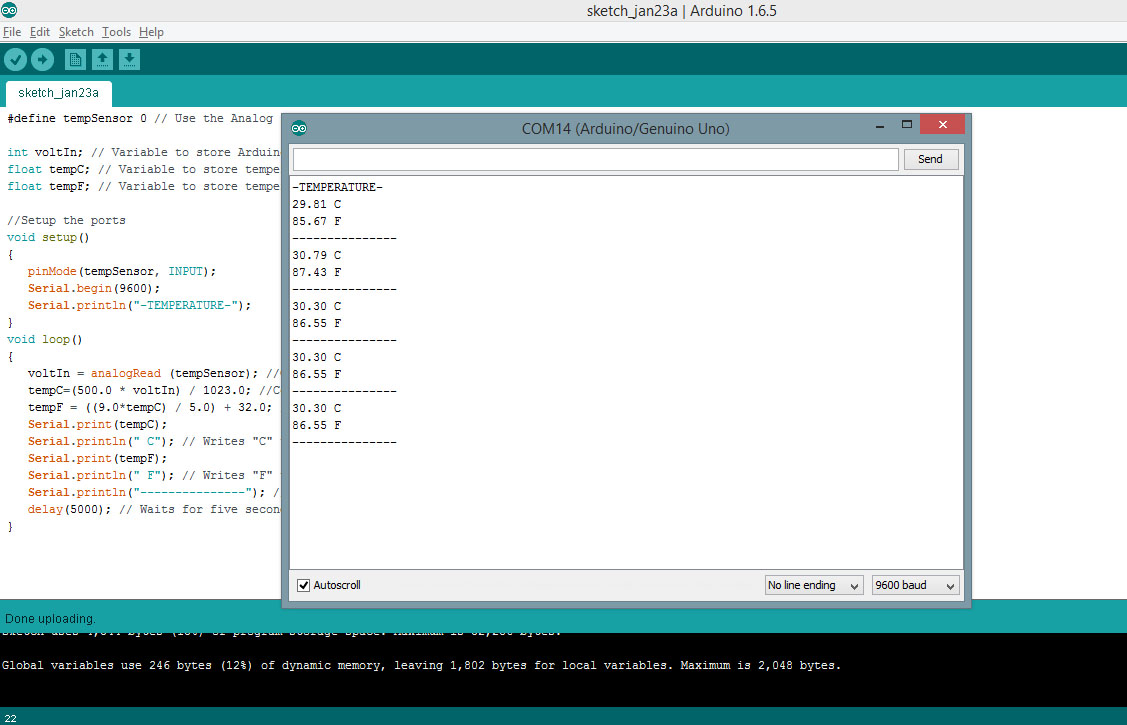# Arduino LM35 Temperature Monitor

Author : Dinesh Kumar Wickramasinghe

Introduction and Images

Hello friends. Temperature sensing using Arduino is very easy. There are several temperature sensors available and in this example I am going to use famous LM35 Temperature sensor. First I will use the Serial monitor to measure the temperature and then use LCD display to monitor the temperature.

Before we begin, here are some images of the completed project.

The LM35 Temperature Sensor.The LM35 series are precision integrated-circuit temperature devices with an output voltage linearly-proportional to the Centigrade temperature. The LM35 device does not require any external calibration or trimming to provide typical accuracies of ±¼°C at room temperature and ±¾°C over a full −55°C to 150°C temperature range. As the LM35 device draws only 60 µA from the supply, it has very low self-heating of less than 0.1°C in still air. The LM35 device is rated to operate over a −55°C to 150°C temperature range.

Connecting LM35 to Arduino Uno.Connect the Pin 1 of the LM35 sensor to the Arduino +5V terminal and Middle pin of the LM35 to Arduino A0 (Analog input 0). Then the third pin of the LM35 to any ground terminal of the Arduino Uno board.

Source code 1 - Using Serial Monitor.

First we are going to monitor the temperature using the Serial Monitor window of the Arduino IDE. Insert the below code to the Arduino IDE and upload it. If you found any error, please double check your code. Now click the Serial Monitor icon of the Arduino IDE. This code will monitor the temperature in every 5 second and report it.

```#define tempSensor 0 // Use the Analog input 0

int voltIn; // Variable to store Arduino A0 pin value
float tempC; // Variable to store temperature in Celcius
float tempF; // Variable to store temperature in Fahrenheit

//Setup the ports
void setup()
{
pinMode(tempSensor, INPUT);
Serial.begin(9600);
Serial.println("-TEMPERATURE-");
}
void loop()
{
voltIn = analogRead (tempSensor); //Command the Arduino to read A0 Analog value
tempC=(500.0 * voltIn) / 1023.0; //Converts volt value to celcius temperature
tempF = ((9.0*tempC) / 5.0) + 32.0; //Converts celcius value to fahrenheit
Serial.print(tempC);
Serial.println(" C"); // Writes "C" to indicate Celcius
Serial.print(tempF);
Serial.println(" F"); // Writes "F" to indicate Fahrenheit
Serial.println("---------------"); //Seperator line
delay(5000); // Waits for five second to read the voltage again
}
```

If you could sucessfully upload the code, You will see an output similar to below screenshot. If you found any error, Double check your connections as mentioned on the above section and also double ckeck the code.Source code 2 - Using LCD Screen.

So this time we are going to connect a LCD screen to our project and create LCD Temperature Monitor. Use the below Schematic to connect LCD screen to your project.Using LCD Screens with Arduino

Insert the below code to Arduino IDE and upload it.

```#include <LiquidCrystal.h>

int voltIn; // Variable to store Arduino A0 pin value
float tempC; // Variable to store temperature in Celcius
float tempF; // Variable to store temperature in Fahrenheit
LiquidCrystal lcd (7, 8, 9, 10, 11, 12); //Initialize the LCD pins

//Setup the ports
void setup()
{
pinMode(tempSensor, INPUT);
lcd.begin(16, 2); // Set the display type 16 * 2
lcd.print("-TEMPERATURE-"); // Display the text on LCD
}
void loop()
{
voltIn = analogRead (tempSensor); //Command the Arduino to read A0 Analog value
tempC=(500.0 * voltIn) / 1023.0; //Converts volt value to celcius temperature
tempF = ((9.0*tempC) / 5.0) + 32.0; //Converts celcius value to fahrenheit
lcd.setCursor(0, 1); //Use the second line of the LCD
lcd.print(tempF); //Print Fahrenheit temperature on LCD
lcd.print((char)223); //Print degree symbol
lcd.print("F "); // Writes "F" to indicate Fahrenheit
lcd.print (tempC); //Print Celcius temperature on LCD
lcd.print((char)223); //Print degree symbol
lcd.print("C"); // Writes "C" to indicate Celcius
delay(5000); //Waits for five second to read the voltage again
}
```

This code will read the temperature in every 5 Seconds and display it on LCD screen. Hope you enjoyed the tutorial. Next time I will update this tutorial to use a Micro SD card and log the temperature to a text file.